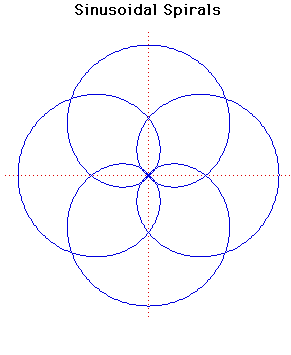# Curves

### Sinusoidal SpiralsPolar equation:
$r^{p} = a^{p} \cos(p \theta )$

### Description

Sinusoidal spirals can have any rational number $p$ in the formula above. Many standard curves occur as sinusoidal spirals.

If $p = -1$ we have a line.

If $p = 1$ we have a circle.

If $p = \large\frac{1}{2}\normalsize$ we have a cardioid.

If $p = -\large\frac{1}{2}\normalsize$ we have a parabola.

If $p = -2$ we have a hyperbola.

If $p = 2$ we have a lemniscate of Bernoulli.

Sinusoidal spirals were first studied by Maclaurin.
They are not, of course, true spirals.

The pedal curve of sinusoidal spirals, when the pedal point is the pole, is another sinusoidal spiral.

The sinusoidal spiral $r^{p} = a^{p} \cos(p\theta)$ inverts to $r^{p} = a^{p}/\cos(p\theta)$ if the centre of inversion is taken at the pole.

### Associated Curves

Definitions of the Associated curves